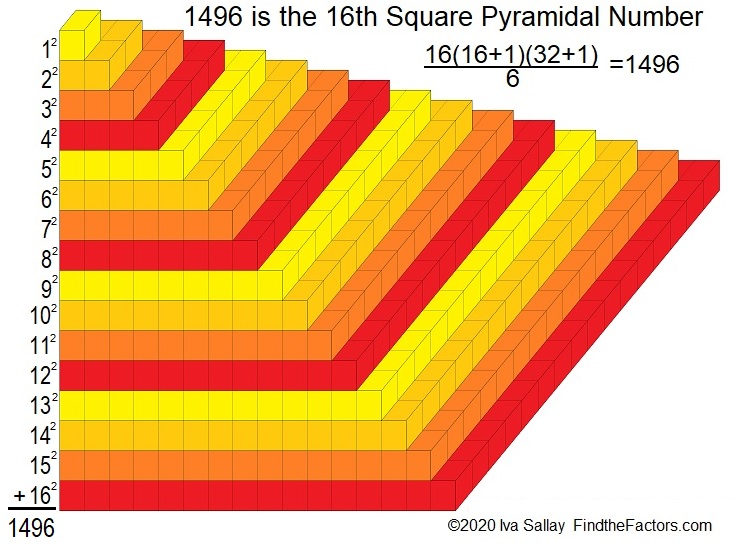# 1496 is a Square Pyramidal Number

Contents

### Visualize 1496 Blocks:

I hoped to make a graphic illustrating that 1496 is the 16th square pyramidal number. I am thrilled that I succeeded!### Factors of 1496:

Knowing the multiplication table and some divisibility tricks helped me find some of 1496’s factors:
96 is 8 × 12, and 4 is even, so 1496 is divisible by 8.
1 – 4 + 9 – 6 = 0, so 1496 is divisible by 11.

• 1496 is a composite number.
• Prime factorization: 1496 = 2 × 2 × 2 × 11 × 17, which can be written 1496 = 2³ × 11 × 17
• 1496 has at least one exponent greater than 1 in its prime factorization so √1496 can be simplified. Taking the factor pair from the factor pair table below with the largest square number factor, we get √1496 = (√4)(√374) = 2√374
• The exponents in the prime factorization are 3,1 and 1. Adding one to each exponent and multiplying we get (3 + 1)(1 + 1) (1 + 1) = 4 × 2 × 2 = 16. Therefore 1496 has exactly 16 factors.
• The factors of 1496 are outlined with their factor pair partners in the graphic below.### Another fact about the number 1496:

1496 is the hypotenuse of a Pythagorean triple:
704-1320-1496, which is (8-15-17) times 88.

This site uses Akismet to reduce spam. Learn how your comment data is processed.